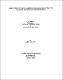## Anodic dissolution of uranium dioxide in simple electrolyte solutions and simulated groundwatersThe anodic dissolution of UO$\sb2$ has been studied in NaClO$\sb4$ NaCl, Standard Canadian Shield Saline Solution (SCSSS) and SCSSS + HCO$\sb3\sp-$ solutions using potentiostatic and cyclic voltammetry techniques. Under potentiostatic conditions two oxidation/dissolution regions were observed. Over the anodic potential range 0 1V $\leq$ E $\leq$ 0.425V (vs. SCE) currents decayed continuously due to the formation of oxidized surface films. The UO$\sb2$ surface was determined to be blocked by a thin film of UO$\sb{2.33}$ and a deposited U$\sp{\rm VI}$ solid distributed uniformly over the surface. In NaClO$\sb4$ and NaCl solutions, the U$\sp{\rm VI}$ solid was a UO$\sb3{\cdot}2$H$\sb2$O deposit. In SCSSS and SCSSS + HCO$\sb3\sp-$ solutions, calcium and silicon ions became incorporated into the U$\sp{\rm VI}$ solid. Over the anodic potential range 0.425V $\leq$ E $\leq$ 0.55V (vs. SCE), the rate of UO$\sb{2.33}$ film formation was equal to the rate of its oxidative dissolution as UO$\sb2\sp{2+}$ (i.e. steady state conditions). In all four solutions, the dissolution/hydrolysis/acidification rate was sufficiently rapid to establish local acidity at high anodic potentials. This local acidity lead to an increase in local solubility of U$\sp{\rm VI}$ which was then transported away from the dissolution sites as UO$\sb2\sp{2+}$ rather than deposited as a U$\sp{\rm VI}$ solid. After anodic oxidation in SCSSS, steady state was established at a higher potential than that for NaClO$\sb4$ and NaCl which indicated that alcium/silicon containing U$\sp{\rm VI}$ phases blocked the oxidative dissolution process until a higher potential was reached. Conversely, after anodic oxidation in SCSSS + HCO$\sb3\sp-$ steady state was established at a lower potential than that for NaClO$\sb4$ and NaCl which indicated that HCO$\sb3\sp-$ accelerated the oxidative dissolution process despite the presence of calcium and silicon ions. (Abstract shortened by UMI.)